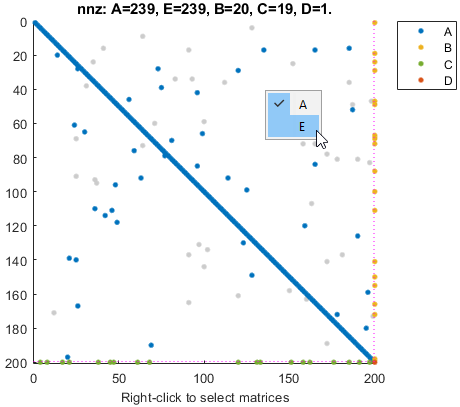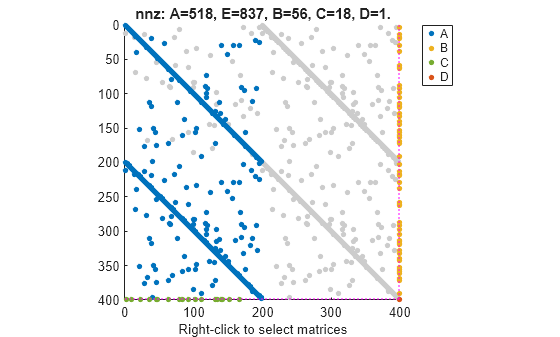このページは前リリースの情報です。該当の英語のページはこのリリースで削除されています。

# spy

`sparss` モデル オブジェクトのスパース パターンを可視化する

## 構文

``spy(sys)``
``spy(AX,sys)``

## 説明

````spy(sys)` は、`sparss` モデル `sys` のスパース パターンをプロットします。プロットには、`sys` の各スパース行列に含まれる非ゼロ要素の数が表示されます。プロットを右クリックして、表示する行列を選択します。```
``spy(AX,sys)` は、ハンドル `AX` をもつ現在の Figure の `Axes` または `UIAxes` オブジェクトにスパース パターンをプロットします。App Designer で `spy` を使用してアプリを作成する場合にこの構文を使用します。`

## 例

すべて折りたたむ

この例では、連続時間スパース 1 次状態空間モデルのスパース行列を含む `sparseFOContinuous.mat` について考えます。

`sparseFOContinuous.mat` からスパース行列を抽出します。

`load('sparseFOContinuous.mat','A','B','C','D','E');`

`sparss` モデル オブジェクトを作成します。

`sys = sparss(A,B,C,D,E)`
```Sparse continuous-time state-space model with 1 outputs, 1 inputs, and 199 states. Use "spy" and "showStateInfo" to inspect model structure. Type "properties('sparss')" for a list of model properties. Type "help sparssOptions" for available solver options for this model. ```

出力 `sys` は、199 の状態、1 つの入力、および 1 つの出力を含む連続時間 `sparss` モデル オブジェクトです。

`spy`コマンドを使用して、`sparss` モデル オブジェクトのスパース性を可視化できます。

`spy(sys)`この例では、離散時間スパース 1 次状態空間モデルのスパース行列を含む `sparseFODiscrete.mat` について考えます。

`sparseFODiscrete.mat` からスパース行列を抽出します。

`load('sparseFODiscrete.mat','A','B','C','D','E','ts');`

`sparss` モデル オブジェクトを作成します。

`sys = sparss(A,B,C,D,E,ts)`
```Sparse discrete-time state-space model with 1 outputs, 1 inputs, and 398 states. Use "spy" and "showStateInfo" to inspect model structure. Type "properties('sparss')" for a list of model properties. Type "help sparssOptions" for available solver options for this model. ```

出力 `sys` は、398 の状態、1 つの入力と 1 つの出力を含む離散時間 `sparss` モデル オブジェクトです。

`spy`コマンドを使用して、`sparss` モデル オブジェクトのスパース性を可視化できます。

`spy(sys)``properties('sparss')`
```Properties for class sparss: A B C D E Scaled StateInfo SolverOptions InternalDelay InputDelay OutputDelay Ts TimeUnit InputName InputUnit InputGroup OutputName OutputUnit OutputGroup Notes UserData Name SamplingGrid ```

## 入力引数

すべて折りたたむ

1 次スパース モデル。`sparss` モデル オブジェクトとして指定します。相互作用または相互接続するコンポーネントがスパース モデル `sys` に含まれる場合は、`spy(xsort(sys))` を使用して基になっているブロック矢印構造を表示します。ブロック矢印構造の詳細については、`xsort` 参照してください。

オブジェクト ハンドル。`Axes` オブジェクトまたは `UIAxes` オブジェクトとして指定します。App Designer で `spy` を使用してアプリを作成するために `AX` を使用します。

R2020b で導入